# My Math Checker

### Try the automatic checker!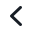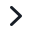Check if the example is correctly calculated

Then replace it with your own calculation.

{ "type": "solutionelement", "name": "solutionelement-checkerdemo", "metadata": {}, "data": { "contents": [{"id": "sdelement-demodata"}], "contentdata": { "sdelement-demodata": { "type": "sdelement", "name": "sdelement-demodata", "metadata": {}, "data": { "dstepdata": { "stask-onlineuser-20190418101022731-387": { "readonly": false, "task": [], "assumption": [], "observation": [], "derivmotivation": [], "term": [ { "text": "\\D\\left(x^3+2x^2+x+1\\right)", "readonly": false, "fillable": false, "attrs": {} }, { "text": "\\D x^3+\\D2x^2+\\D x+\\D1", "readonly": false, "fillable": false, "attrs": {} }, { "text": "3x^2+2\\cdot2x+1+0", "readonly": false, "fillable": false, "attrs": {} }, { "text": "3x^2+4x+1", "readonly": false, "fillable": false, "attrs": {} } ], "relation": [ { "text": "=", "readonly": false, "fillable": false, "attrs": {} }, { "text": "=", "readonly": false, "fillable": false, "attrs": {} }, { "text": "=", "readonly": false, "fillable": false, "attrs": {} } ], "motivation": [ { "text": "use the sum rule to separate the terms", "readonly": false, "fillable": false, "subshidden": false, "subderiv": [], "attrs": {} }, { "text": "calculate the derivative of each term", "readonly": false, "fillable": false, "subshidden": false, "subderiv": [], "attrs": {} }, { "text": "simplify", "readonly": false, "fillable": false, "subshidden": false, "subderiv": [], "attrs": {} } ] } }, "dsteps": [ { "id": "stask-onlineuser-20190418101022731-387", "type": "task" } ] } } } } }

# Derivatives

Check if the derivatives example to the left is calculated correctly. You can exchange it with your own calculation, and check that one as well.

# Use the checker always when practicing math

THEORY How to solve derivatives?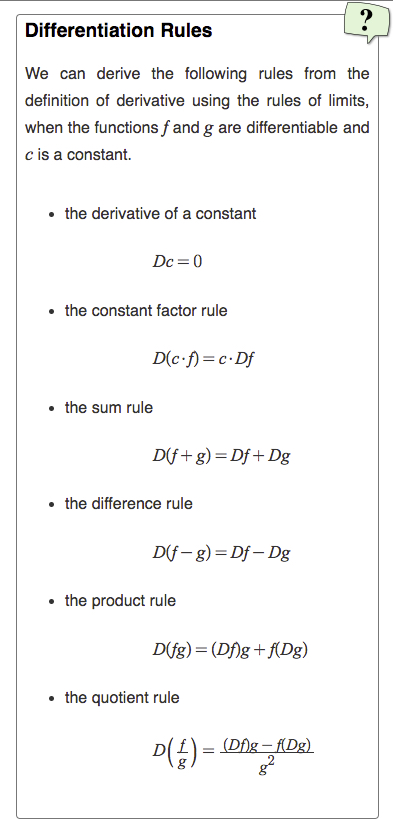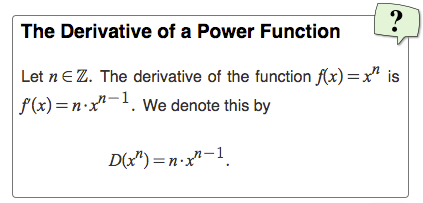### Checking for Middle School, High School and University Level Math

Whether you are a middle school, high school or university level student, or an adult refreshing your memory, 4f Checker helps you to check if your derivation is mathematically correct.

The checker formulates each step in the derivation as a mathematical theorem, and sends the theorem to an automatic theorem prover that runs in the cloud. The derivation is correct, if each step in the derivation is proved correct.

### Trust yourself

In math, there are many ways to get to a solution. Math operations can be made in different order, calculations in several or few steps. We all think in different ways, and it may be difficult to see it the way your teacher does and teaches you. This doesn't mean that your way is wrong. With the checker you can solve assignments your way, and check if it is mathematically correct.

### Subscription details

The monthly subscription My Math Checker includes digital notebooks where you can calculate all your assignments and check the correctness of your calculations. The checker goes through the solution step by step and points out the weak spots and potential problems in your mathematical arguments.

My Math Checker subscription gives you

• Math tools for writing math easily on the computer
• Embed videos and material
• Step-by-step checking of your calculations
• 200 checks / month subscribed

### Example assignments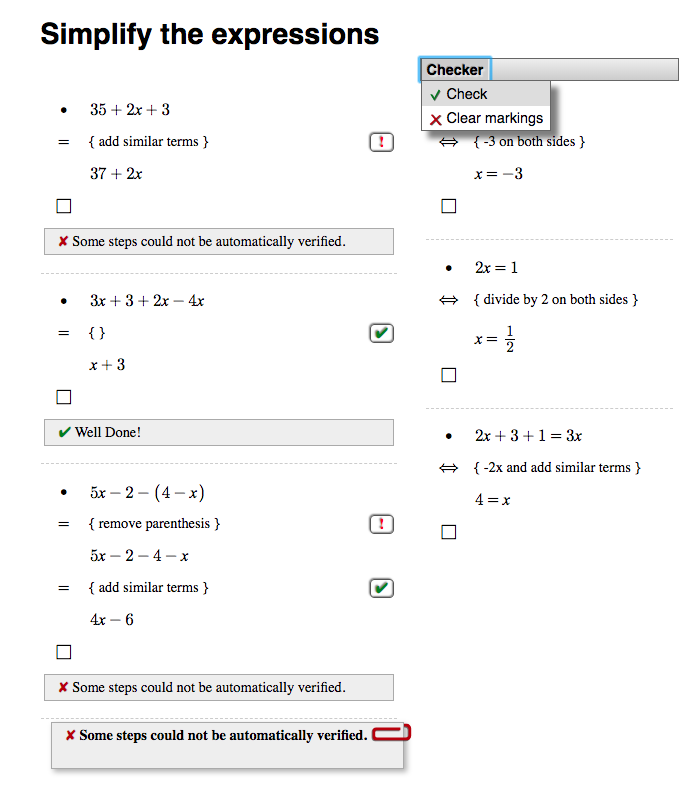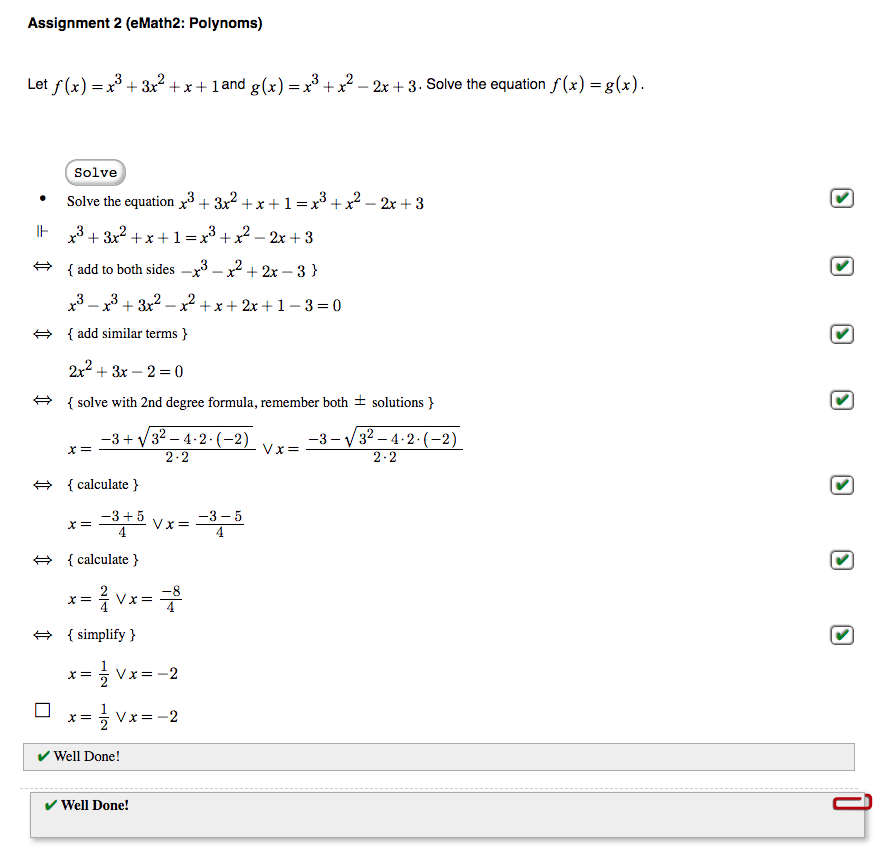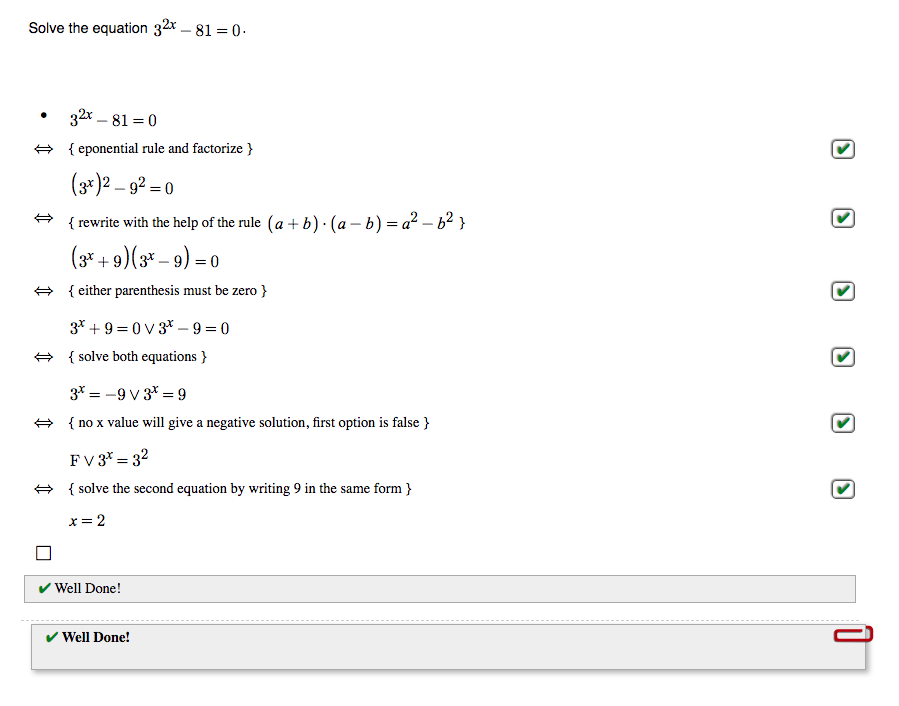Let the computer check your math solution, step by step.
The checker marks all steps that need attention, so that you can correct your solution while you are working on it
News update

We are excited to announce that we have added a CAS Calculator to 4f Checker. This means that you can now both check your solutions as before, but also calculate intermediate values, perform trigonometric and logarithmic operations and solve equations with the calculator. This feature will be automatically available for our existing 4f Checker users.